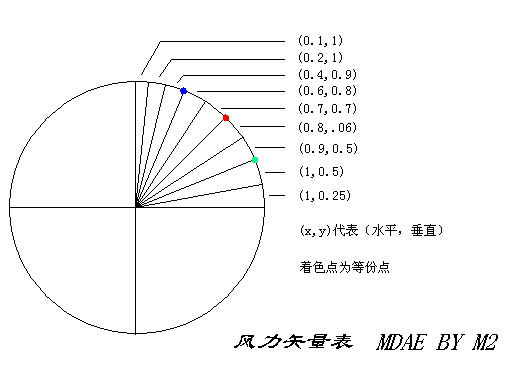from soyabean:

m2's shiliang calculations
Hey, im eating dinner now.. nothing to do, translate the shiliang calculations for u guys ^^ First of all, this is an introduction to shiliang, because there are more styles to it, i will update it when i have time. In gb, there are 2 methods of calculations known to me. One is the wind index method that most of us are familair with. The other, is shiliang. Shiliang deals with the horizontal and vertical displacement due to the wind effect wheres wind index resolves these 2 displacement into a factor. The origins and details of shiliang can be found at knat's forums, in the original m2 guide.

Next, based on these 2 methods, a few styles of calculation has been derived. They are mainly these 3, fix power, fix angle, change angle change power. Some examples of fix power calculations include the xiaopao, banpao, and man pao. And the change angle change power calculations are the Bian Li Suan Jiao formulas.

Below, im going to translate the introduction part for m2's guide, which deals with the second style of calculation, fix angle.

All of us know that it is impossible to make a complete wind chart based on wind index for fix angle, for 2 simple reasons. Firstly, effect varies with distance, secondly, effect varies with wind. Therefore, shiliang becomes a more effective way of calculation.

Style: fix angle, calculate power

Wind chart:Screen: 20 parts
x: horizontal displacement
y: vertical displacement
d: change in power
f: power at wind 0
a: angle

This formula uses armor as a reference. (all other bots can be used the same way except for mage and boomer, for boomer, just x2 the result)

Armor 60:

formula: 2x+y/100

Armor 40

formula: x+y/100

Complete formula: D=F*(Y+Xtga)/2(100-Y+Xtga)
Recommended formula: D=(Y+X)/(100-Y+X) for angle 40, D=(Y+2X)/(100-Y+2X) for angle 60

Explaination for simplification of the complete formula:
D=F*(Y+Xtga)/2(100-Y+Xtga)

Taking angle to be 40 (tga = 1), therefore,
D=F*(Y+X)/2(100-Y+X)

Taking power to be 2.0, therefore,
D=(Y+X)/(100-Y+X)

Usually, -Y+Xtga tends to be very small (there are exceptions, and they will be discussed below). Hence, we can further simplify it to become:
D=(Y+X)/(100)

When angle becomes 60, tga=2. Thats how m2 derived 2x+y/100

Example:
I will use angle 60 as example, using simplified formula: 2x+y/100
opponent 14 parts away. power to use at wind 0=2.0
wind blowing at bearing of 045, 10
formula = 2x+y/100
=2(0.7*10)+(0.7*10)/100
=0.21
power to use = 2.0 - 0.21 = 1.79 ~ 1.8

And now for the most important part, how to reduce errors. this formula has been simplified alot to allow easy calculations.

1)The change in power due to wind is only relevant for distance of 2.0 power, which is 14 parts.(We took F=2.0 previously to simplify the equation) If distance becomes further, like 1 screen(20 parts), the change in power increases. the reverse is true. u can either feel for the difference, or u can add some calculations, just take b/2*result. b being the power required to reach the distance. for full screen, b will be 2.45.

2)Secondly, a few wind directions have weird effect. (when 100-Y+2Xtga becomes very big or very small). For example, wind blowing at a bearing of 315, 20, 100-Y+2Xtga for angle 60 = 100 - (0.7*20) + (-0.7*20*2) = 58. You will need to multiply the result by some wind index to change the ans. Wind blowing around 000, 270, 315, *1.4 to result. Wind blowing around 090, 135, 180, *0.8 to result.

With that, the general idea of shiliang has been established. when i have time, i will translate the use of shiliang in xiaopao (2.4) banpao (2.8-2.95-3.05) for armor ^^

* If u are using the recommended formulas, u can ignore the second way of reducing errors.

Power at wind 0 for armor 40.

4 ————1.0
5 ————1.1
6 ————1.2
7 ————1.3
8 ————1.4
9 ————1.5
10————1.6
11————1.7
12————1.8
13————1.87
14————1.95
15————2.02
16————2.1
17————2.17
18————2.25
19————2.3
20————2.35

Armor 60 u can find it somewhere on this forums already. This one is provided by m2, so i suppose you will measure from the center of ur bot to where u want to hit.

Style: Fix power calculate angle

Manpao:
Firstly, just for your information, the calculations for this type of shooting style still contains some errors. It is not recommended to use it. m2 provided calculations for both boomer and armor, but I will only translate armor as this method for boomer is very impractical.

Power: Full power
Screen: 11 parts

Formula
Angle: Power at wind 0 + Horizontal displacement + Vertical displacement

Horizontal displacement = X/10
Vertical displacment = Y/2

Example:
Wind blowing at a bearing of 045, 20.
Distance = 1 screen
Angle: 79 + (0.7*20/10) + (0.7*20/2) = 79 + 1.4 + 7 = 87.5

Xiaopao and Banpao:
Known to many of us, xiaopao and banpao can be used with 14 parts, 20 parts, and 30 parts. But since 14 parts is not very practical, unless u r shooting beyond 1 screen distance, I will only translate for 20 parts and 30 parts.

Xiaopao:
Power: 2.4
Screen: 30 parts

Formula
Angle = Angle at wind 0 + Horizontal displacement + Vertical displacment

Horizontal displacement = X/2 (When X/2 is clsoe to 5, add 1 angle)
Vertical displacement = Y/8 (This is accurate for halfscreen - 1 screen distance, if distance is less than half, u will use Y/4, and as distance increases to 1-1.5 screen, use Y/16)

Example:
Wind blowing at the bearing of 079, 15
Distance: 24 parts
Angle = (90-24) + [(1*15/2)+1] + (0.5*15/8 ) = 75

Tired... later den translate banpao